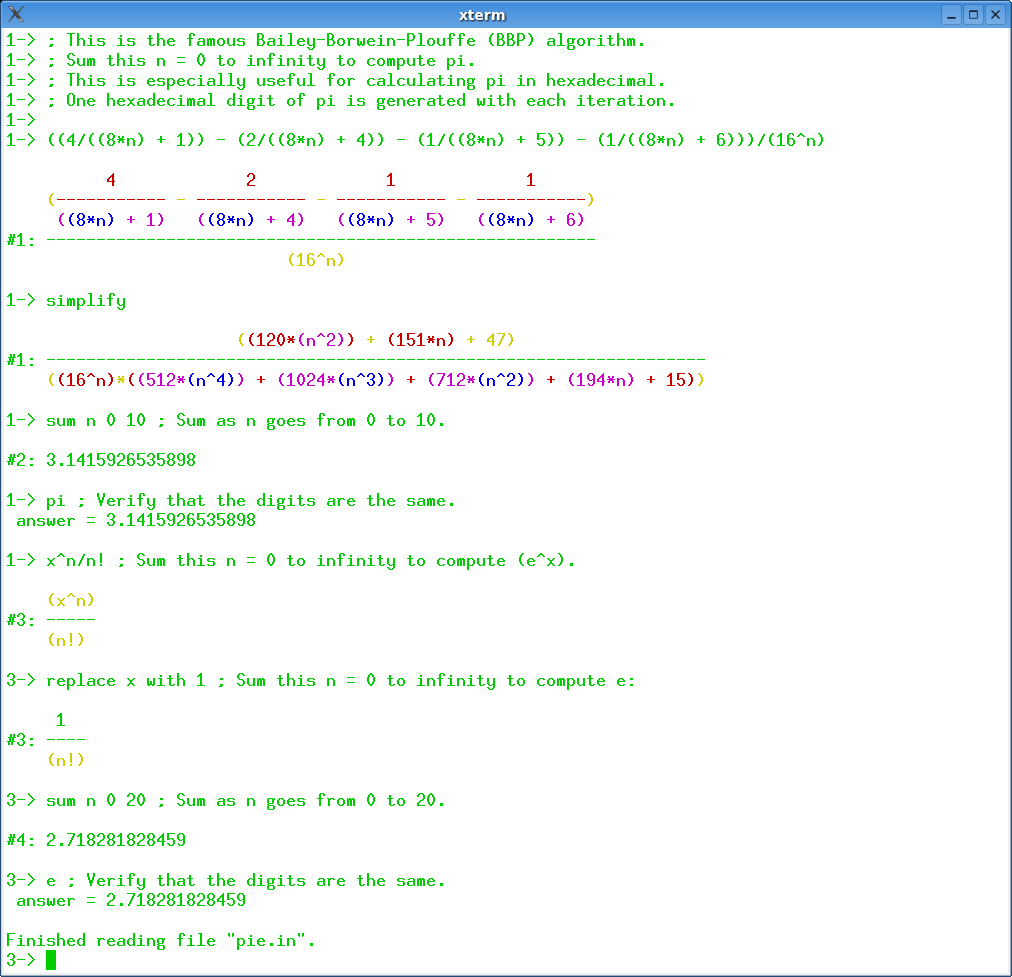## 13 Search Results

### Axiom

Axiom is a general purpose Computer Algebra system. It is useful for research and development of mathematical algorithms. It defines a strongly typed, mathematically correct type hierarchy. It has a programming language and a built-in compiler.

### CoCoA

CoCoA is a system for Computations in Commutative Algebra. It is able to perform simple and sophisticated operations on multivaraiate polynomials and on various data related to them (ideals, modules, matrices, rational functions). For example, it can readily compute Grobner bases, syzygies and minimal free resolution, intersection, division, the radical of an ideal, the ideal of zero-dimensional schemes, Poincare' series and Hilbert functions, factorization of polynomials, toric ideals. The capabilities of CoCoA and the flexibility of its use are further enhanced by the dedicated high-level programming language. For convenience, the system offers a textual interface, an Emacs mode, and a graphical user interface common to most platforms.

### Fermat

Fermat is a super calculator - computer algebra system, in which the basic items being computed can be rational numbers, modular numbers, elements of finite fields, multivariable polynomials, multivariable rational functions, or multivariable polynomials modulo other polynomials. Fermat is available for Mac OS, Windows, Unix, and Linux. It is shareware. The basic “ground ring" F is the field of rational numbers. One may choose to work modulo a specified integer n, thereby changing the ground ring F from Q to Z/n. On top of this may be attached any number of unevaluated variables t_1, t_2, .. t_n., thereby creating the polynomial ring F[t_1, t_2, .. t_n] and its quotient field, the rational functions. Further, polynomials p, q, .. can be chosen to mod out with, creating the quotient ring F(t_1, t_2, ..)/[p, q, ...]. It is possible to allow Laurent polynomials. Once the computational ring is established in this way, all computations are of elements of this ring.

### GAP

GAP is a system for computational discrete algebra, with particular emphasis on Computational Group Theory. GAP provides a programming language, a library of thousands of functions implementing algebraic algorithms written in the GAP language as well as large data libraries of algebraic objects. GAP is used in research and teaching for studying groups and their representations, rings, vector spaces, algebras, combinatorial structures, and more. GAP is developed by international cooperation. The system, including source, is distributed freely under the terms of the GNU General Public License. You can study and easily modify or extend GAP for your special use. The current version is GAP 4, the older version GAP 3 is still available.

### Givaro

In the joint CNRS-INRIA / INPG-UJF project APACHE, Givaro is a C++ library for arithmetic and algebraic computations. Its main features are implementations of the basic arithmetic of many mathematical entities: Primes fields, Extensions Fields, Finite Fields, Finite Rings, Polynomials, Algebraic numbers, Arbitrary precision integers and rationals (C++ wrappers over gmp) It also provides data-structures and templated classes for the manipulation of basic algebraic objects, such as vectors, matrices (dense, sparse, structured), univariate polynomials (and therefore recursive multivariate). It contains different program modules and is fully compatible with the LinBox linear algebra library and the Athapascan environment, which permits parallel programming.

### Mathomatic

Mathomatic is a free, portable, general-purpose CAS (Computer Algebra System) and calculator software that can symbolically solve, simplify, combine, and compare equations, perform complex number and polynomial arithmetic, etc. It does some calculus and is very easy to use.

More information### Matlab

MATLAB is a high-level language and interactive environment that enables you to perform computationally intensive tasks faster than with traditional programming languages such as C, C++, and Fortran.

### Maxima

Maxima is a system for the manipulation of symbolic and numerical expressions, including differentiation, integration, Taylor series, Laplace transforms, ordinary differential equations, systems of linear equations, and vectors, matrices, and tensors. Maxima produces high precision results by using exact fractions and arbitrarily long floating point representations, and can plot functions and data in two and three dimensions.

MuPAD is a mathematical expert system for doing symbolic and exact algebraic computations as well as numerical calculations with almost arbitrary accuracy. For example, the number of significant digits can be chosen freely. Apart from a vast variety of mathematical libraries the system provides tools for high quality visualization of 2- and 3-dimensional objects. On Microsoft Windows, Apple Macintosh and Linux systems, MuPAD offers a flexible notebook concept for creating mathematical documents combining texts, graphics, formulas, computations and mathematical visualizations and animations. On Microsoft Windows MuPAD further supports the technologies OLE, ActiveX Automation, DCOM, RTF and HTML. Thus it offers a natural integration in Office applications like Word or PowerPoint as well as others.

### NTL

NTL is a high-performance, portable C++ library providing data structures and algorithms for manipulating signed, arbitrary length integers, and for vectors, matrices, and polynomials over the integers and over finite fields.

### Octave

GNU Octave is a high-level language, primarily intended for numerical computations. It provides a convenient command line interface for solving linear and nonlinear problems numerically, and for performing other numerical experiments using a language that is mostly compatible with Matlab. It may also be used as a batch-oriented language. Octave has extensive tools for solving common numerical linear algebra problems, finding the roots of nonlinear equations, integrating ordinary functions, manipulating polynomials, and integrating ordinary differential and differential-algebraic equations. It is easily extensible and customizable via user-defined functions written in Octave's own language, or using dynamically loaded modules written in C++, C, Fortran, or other languages.

More information